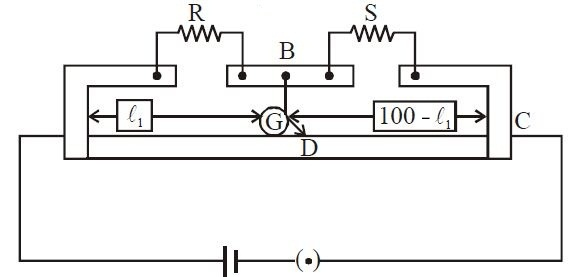# Meter Bridge-1!

In a meter bridge, point $D$ is neutral point as shown in the figure.Which of the following statements is correct

(A) The meter bridge cannot be configured to have any other neutral point for this set of resistances.

(B) When the jockey contacts a point on meter wire left of $D$, current flows to $B$ from the wire.

(C) When the jockey contacts a point on the meter wire to the right of $D$, current flows from $B$ to the wire through galvanometer.

(D) When $R$ is increased, the neutral point shifts to left.

×

Problem Loading...

Note Loading...

Set Loading...